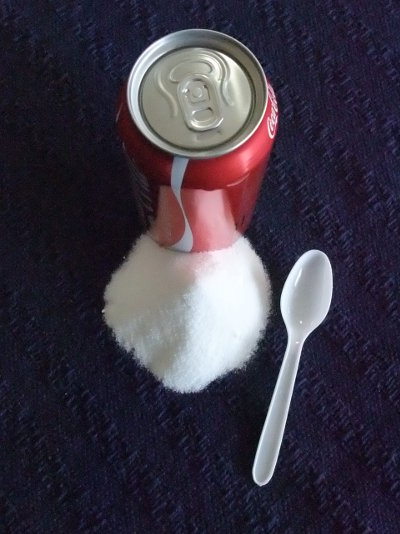# Operations With Whole Numbers

Whole numbers include the positive counting numbers 1, 2, 3 and so on as well as zero and all the negative numbers –1, –2, –3 etc.

The mathematical name for whole numbers is integers.

Mathematical operations are addition, subtraction, multiplication and division. Some of the words that we use for these operations include:

• Addition - sum, more than, total, amount altogether
• Subtraction - difference, less than, amount left, remainder
• Multiplication - product, total, amount altogether, lots of, of
• Division - quotient, shared number, per

## Example One - Addition

What is the sum of 345 and 678? (Line up the columns.)

 345 + 678 1023

## Example Two - Subtraction

What is the difference between 678 and 345? (Line up the columns.)

 678 – 345 333

## Example Three - Multiplication

What is 64 × 25? (Multiply by 5 first, and then multiply by 20 which is 10 × 2.)

 64 × 25 320 + 1280 1600

## Example Four - Division

Share 180 amongst 15 people.

 12 15 180 –15↓ 30–30 0

## Question - How many teaspoons of sugar are in a can of cola?The label of a 375 ml can of cola drink states that it contains 40 grams of sugar. One teaspoon contains about 5 grams. How many teaspoons of sugar is this?

8

## Maths Fun

Calculate these. What's the pattern? How do they work?

1 × 1 =
11 × 11 =
111 × 111 =
1111 × 1111 =
11111 × 11111 =

1 × 8 + 1 =
12 × 8 + 2 =
123 × 8 + 3 =
1234 × 8 + 4 =
12345 × 8 + 5 =